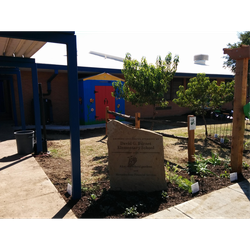Author:
Out Teach
Subject:
Mathematics
Material Type:
Lesson Plan
Level:
Lower Primary, Upper Primary
3
Tags:
• Math
• Operations and Algebraic Thinking
• Out Teach
• Variable
Creative Commons Attribution Non-Commercial Share Alike
Language:
English
Media Formats:
Text/HTML

# Algebraic Reasoning -- Out Teach## Overview

Students work with the concept of equality and balance to solve algebraic equations.

# Background for Teachers:Students should have prior knowledge of some algebraic ideas, and they are ready to explore these and other algebraic concepts and skills. Using objects, they collect outdoors for variables it is much easier than drawing geometric shapes.

Students have great interest in solving algebraic problems because they like the mystery of working with unknowns and having to reason their way to the solution.

• Equations

• Variable

• Equal sign

• Balance

# Guiding Question:

Which value(s) will make an equation true?

# Engage:

Write down 2 equations (1 correct and 1 incorrect).

Tell students to brainstorm with their partner which of the equations is correct.

Students share out ideas on how they identified the correct equation.

Share with students that they will be creating equations using plants or objects from the garden.

# Explore:

Ask students to move around the outdoor classroom and choose at least 20 different objects.

After students have collected their 20 objects (it is okay if they do not have exactly 20), bring them back to the central gathering area.

The teacher should also collect a sample of objects for modeling.

# Explain:

Once all students are back, model how to take inventory of the objects they collected by creating a key/variable of the objects. Each object needs to be represented by letters:

X= number of leaves collected (8)

Z= number of rocks collected (7)

A= number of sticks collected (5)

B= number of flowers collected (9)

Model for the students how to create an equation using the key/variable:

y = leaves + rocks

y = x + z

15 = 8 + 7

Questions to assess their thinking:

• What is happening on each side of the equation?

• What variable are we using to represent the leaves, rocks, etc.?

• Can you represent the same thing using a different operation?

• Why must we do to keep both sides of the equation balanced?

Share with students that the equal sign indicates that the expressions on both sides, must have the same value when you simplify them.

# Elaborate:

Allow students to create at least 5 equations using the objects they found.

Encourage students to work together, combining their objects to create their key and corresponding equations.

# Evaluate:

Invite students to create equations for a partner to solve. (For example, 15 = 8 + Z, so how many rocks were collected?)

# Extension:

Once students have learned about equations with brackets and parentheses, have them create new equations from the number of objects they found.